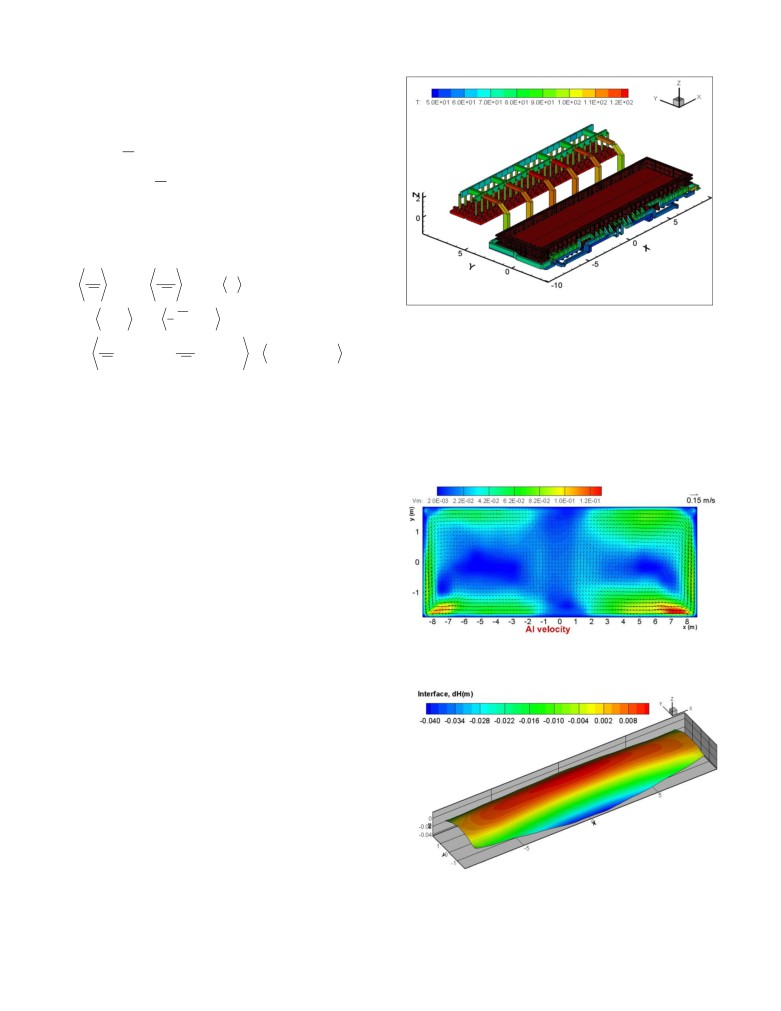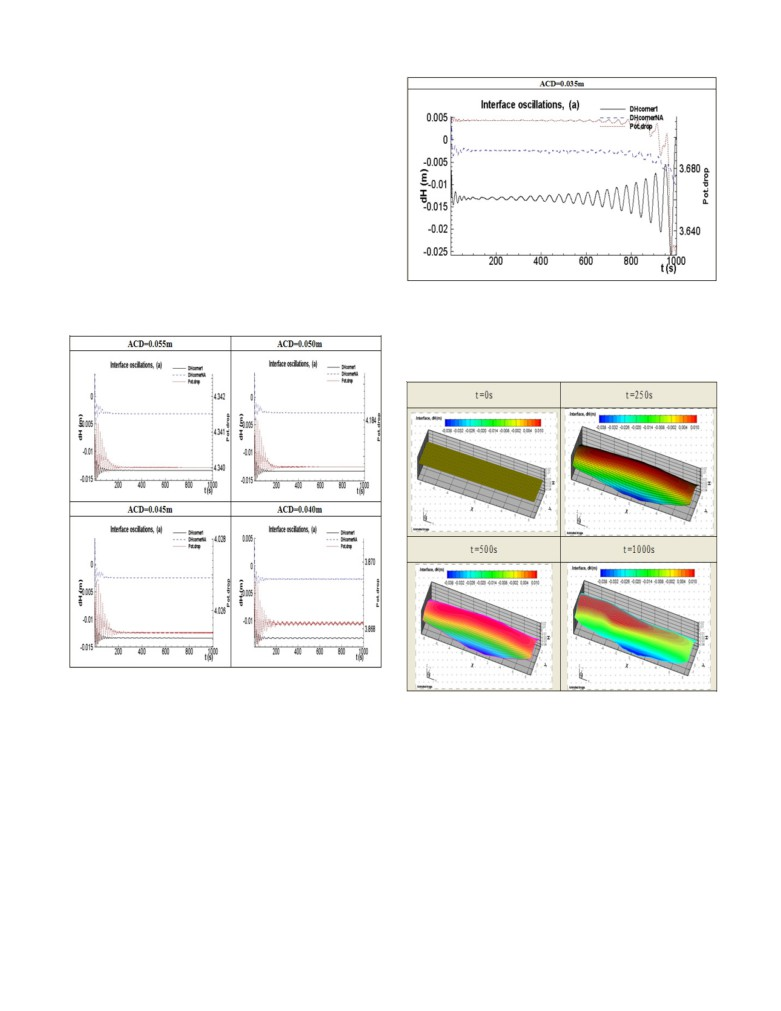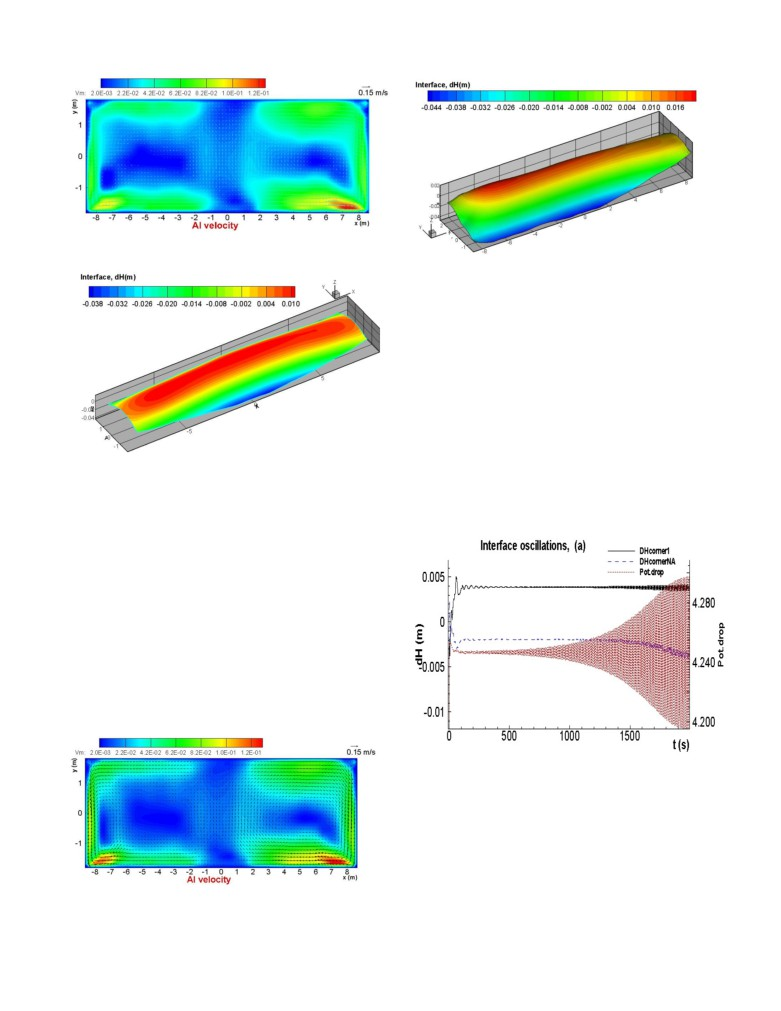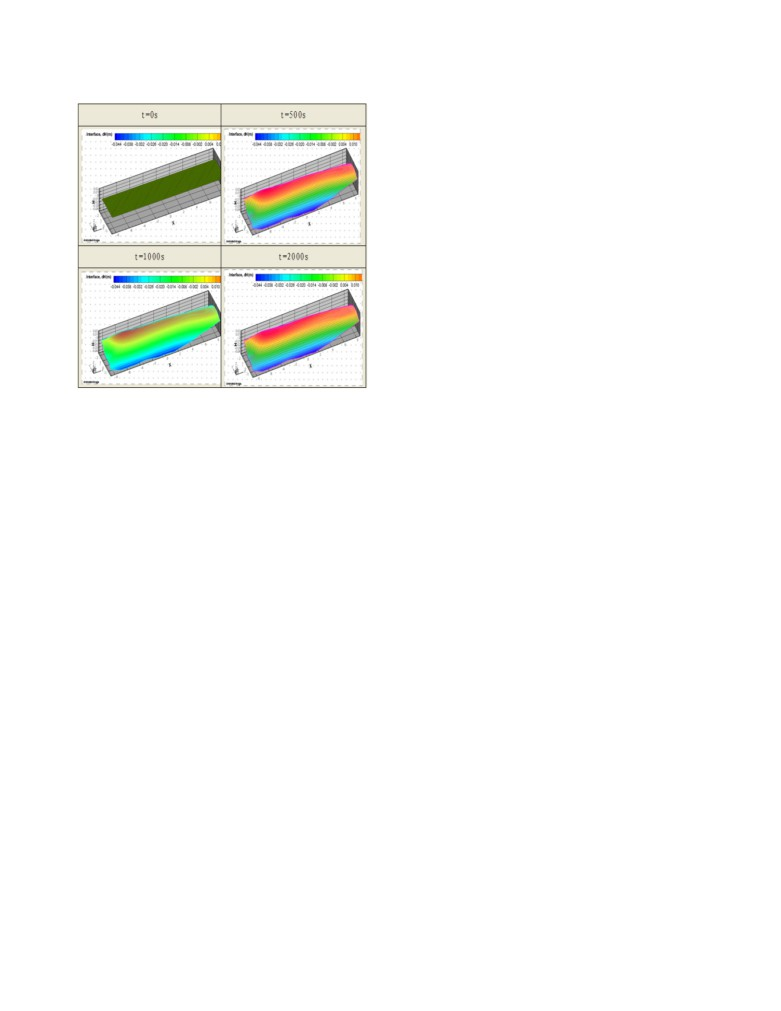PRODUCTION APPLICATION STUDY ON MAGNETO-HYDRO-DYNAMIC STABILITY
OF LARGE PREBAKED ANODE ALUMINUM REDUCTION CELL
Ruan Shaoyong1, Yan Feiya1, Marc Dupuis2, Valdis Bojarevics3, Zhou Jianfei1,
1CHALIECO GAMI Guiyang Guizhou China 550081
2GéniSim Inc., 3111 Alger St., Jonquière, QC, Canada, G7S 2M9
3University of Greenwich, School of Computing and Mathematics
30 Park Row, London, SE10 9LS, UK
Keywords: aluminum reduction cell; magneto-hydro-dynamic stability.
Abstract
This paper introduces the MHD stability theory of pot, and
The magneto-hydro-dynamic stability of an aluminum reduction
establishes the 3-dimensional calculation model of MHD stability
cell has an important influence on aluminum electrolysis
of pot as per actual pot dimension and busbar arrangement, as
production. The paper introduces the research theory of magneto-
well as compares the calculation against observations in one
hydro-dynamic stability of a cell and puts forward the concepts of
certain smelter operating 340kA pots in China.
“stationary state” and
“transient state” of a reduction cell. A
magneto-hydro-dynamic stability software is then used to
Magneto-hydro-dynamic stability theory
calculate two different cell conditions. The calculated results
prove to be consistent with the actual production, which confirms
Definition of pot production states
the model validity.
The large prebaked anode aluminum reduction pot can be
Introduction
mathematically represented into
2 states under production
conditions. One is called “stationary state”, namely the pot is
Aluminum reduction pot consists of carbon anode, bath melt,
under non-disturbance conditions with stable current and without
metal melt and carbon cathode. Large DC passes through the
anode change, tapping, anode effect (AE) and breaking feeding.
aluminum busbar, the anode, the bath, the metal and the cathode
Such state is a kind of ideal state, that never really occur during
etc. during aluminum reduction, which generates hundreds of
actual production, generally pots are continuously disturbed by
Gauss strong magnetic field, and the magnetic field in the pot
normal process operations. The other one is called
“transient
interacts with the current in the metal to generate the
state”, namely the pot is under disturbance conditions with current
electromagnetic force which accelerates the metal circulation in
fluctuation, anode change, tapping and breaking feeding which
the pot, with the result that on one hand it is an efficient way to
can not be prevented. In short, “stationary state” is a state without
dissolve alumina in the bath and then reduce it to aluminium, but
any temporal disturbances, and “transient state” is a state where
on the other hand causes metal pad fluctuation, so that the current
temporal disturbances are present.
efficiency (CE) is reduced and the energy consumption must be
increase in order to prevent excessive metal pad fluctuation. In
Mathematic definition of MHD stability of pot
severe cases, the metal can splashes from the pot to cause
accident.
For the “shallow water” approximation the horizontal dimensions
Lx and Ly are assumed to be much larger than the typical depth
The study on the conditions that generate metal pad fluctuation
H, and the interface wave typical amplitude A is assumed to be
caused by the magnetic force in the pot are called magneto-hydro-
small relative to the depth.
dynamic (MHD) stability studies in the aluminum industry. With
the development of large reduction pot, the pot capacity gradually
With the purpose to derive weakly nonlinear shallow layer
increases, so the MHD stability has become the core issue in the
approximation Boussinesq equations for the wave motion we will
large pot design, as well as the important index reflecting the
need to estimate the terms in the full three dimensional Navier-
merits of pot design. Generally the pot having good MHD
Stokes equations of motion. Nondimensional fluid flow equations:
stability is characterized by better busbar arrangement, better
continuity, horizontal momentum and vertical momentum
magnetic field distribution, low voltage fluctuation noise, and
transport, are respectively[2,3,4,5]:
better CE and power consumption index.
1
u
+δ
w =0
(1)
k k
z
Therefore, MHD stability studies has practical significance,
1
analysing the various input conditions and physical parameters
u
+u
u δ
w
u
=
t
j
k k j
z
j
that are affecting the pot stability performing mathematical
1
2
simulation of the metal pad fluctuation affected by the pot design
p+Re
(δ
ν
u +
ν
u
)+E f
(2)
j
z e z j
k e k
j
j
and process operation. Researchers have kept exploring and
1
perfecting the calculation method of the MHD stability for years
w+uw
+δ
w
w=
t
k
k
z
in aluminum industry, yet it seems from the published literature
1
1
2
1
that there are only few experts and scholars who can successfully
δ
p+Re
(δ
ν
w+ν
w)+E
f
δ
(3)
z
z e z
k e
k
z
solve the issue of MHD stability calculation.
1When the depth averaging procedure is applied to the horizontal
momentum equations (2) we obtain:
u
+u
u
=
t
j
k
k
j
1
p(H
) ε∂ ς
µu
+Re
ν
u
(4)
j
0
j
j
k e
k
0
j
1
2
2
+
Ef
δEH
f
+O(ε
,δ
,εδ )
j
2
i
j
0z
The momentum (4) and continuity (1) equations for the two fluid
layers can be combined in a single nonlinear wave equation for
the interface ζ(x,y,t).
ρ
µρ
ε
ς +
ε
ς +ε ρ
ς
=
tt
t
jj
H
H
1
(5)
E f
δE
H
f
j
j
2
jj
z
Figure 1. Model of 340 kA pot including busbar layout
ρ
µρ
ε
(ςu
)
+
(ςu
)
ρ∂
(u
u
)
tj
jo
j
jo
j
k
k
j
Initial “stationary” state
H
H
The equation (5) is used for the numerical solution of the interface
Before solving in full non-linear transient mode, it is
waves development with coupling to the horizontal circulation
advantageous to solve first the “stationary” state in order to be
obtained from the numerical solution of (4).
able to screen out quickly less promising design based on some
design criteria like the maximum vertical component of the
The equations are by definition transient but depending on the
magnetic field (Bz), maximum horizontal current component in
initial conditions and in the absence of further perturbations could
converge to a “stationary state” where the solution is no longer
deformation of the metal surface per example.
changing when time pass. That “stationary state” solution can be
characterized with high or low
deformation and by high or low horizontal circulation flow.
Physical definition of pot MHD stability
It concludes that from the above-mentioned arguments the study
on the pot MHD stability should have 2 objectives: 1. quickly
study the
“stationary state” solution trying to identify
characteristics of a stable design; 2. generate a perturbation and
carry a much longer fully non-linear “transiant state” analysis to
really check if the cell design is predicted to return on its
“stationary state” after such a perturbation.
Figure 2. Initial “stationary” state metal flow under ACD
In summary, the issue of pot MHD stability can be defined as
= 0.045m
follow: disturbances happen on the pot under normal production
conditions or in some special conditions like when some anodes
are removed, the pot can then return the its original state or transit
to the new stable state after certain time. If it does, the pot is
regarded as stable under such normal production state or special
conditions, otherwise it is regarded as unstable.
Calculated results under normal conditions
Calculation model of pot and busbar layout
Using the MHD-Valdis computer software, the relevant
parameters regarding pot and busbar layout are input it according
to a particular form, thus obtains a model of 340KA pot and
busbar layout in a aluminum smelter. The resulting geometry
setup of the model is shown in figure 1.
Figure 3. Initial “stationary” state metal surface under
ACD = 0.045m
2Voltage fluctuation result chart
As we can see in figures 2 and 3, the initial “stationary” state of
the
340 kA pot looks quite acceptable with a low velocity
symmetric recirculation flow pattern (maximum of 12 cm/s) and a
symmetric and acceptable maximum surface deformation (less
than 5 cm). Unfortunately, solving only the initial “stationary”
state is not enough to know if the pot will be stable in operation,
for that the full non-linear transient solution must be solve as well.
The pot voltage fluctuation after an initial perturbation is
calculated under different anode-cathode distances
(ACD)
including 0.055m, 0.05m, 0.045m and 0.040m. The results are
presented in figure 2 for 3 variables including corner anode ACD
on the downstream duct end, corner anode ACD on the upstream
at tapping end the total pot voltage are selected. Pot voltage
fluctuation resulted from metal fluctuation is observed for that
Figure 5. Voltage fluctuation chart under ACD = 0.035m
whether it becomes stable as time goes on.
Metal surface fluctuation chart
As time goes on, under ACD 0.035m the metal surface fluctuation
chart is as follows:
Figure 4. Voltage fluctuation chart under ACD between
0.040m and 0.055m
Figure 6. Metal surface fluctuation chart under ACD
It shows that the pot can remain stable as the ACD reduces to
= 0.035m
0.040m, but if the voltage fluctuation increases, the conditions
reflected in the production are that the noise increases.
It shows clearly that the metal surface fluctuation is out of control
broadly and the metal could eventually splashed out of the pot to
In order to make distinction and comparison, the voltage
cause an accident.
fluctuation under ACD 0.035m is calculated.
Final “stationary” state
It shows that as time goes on the metal fluctuation increases
Notice that if the pot is predicted to be stable, “transient” state
gradually under the corner anode on the downstream duct end
under ACD 0.035m, thus brings out the much voltage fluctuation
triggered by the perturbation will
“converged” back into a
of the total pot, which may generate short-circuit risk. Therefore
“stationary” state that will by definition no longer evolves as time
this illustrates the pot is impossible to remain stable under ACD
pass.
0.035m.
3Figure 7. Final “stationary” state metal flow under ACD
= 0.045m
Figure 10. Initial “stationary” state metal surface under
ACD = 0.045m
As we can see comparing figures 2 and 3 with figures 9 and 10,
the initial “stationary” state metal flow is not much affected by the
“permanent” removal of the 2 anodes but the initial “stationary”
state metal surface is quite affected as the metal surface
deformation is no longer symmetric and the maximum
deformation has increased to 6 cm.
Voltage fluctuation result chart
So clearly removing anodes is not good for the pot MHD stability
but again only by running the full non-linear transient analysis
will we know if the pot will remain stable under those special
Figure 8. Final “stationary” state metal surface under
conditions. Under ACD 0.045 m, with 2 corner anodes removal
ACD = 0.045m
on downstream duct end, the pot voltage fluctuation chart is as
follows:
As we can see comparing figures 2 and 3 with figures 7 and 8,
after being affected by the perturbation, the pot is predicted to
settle back into the initial “stationary” state which may not be
systematically the case.
Calculated results with some anodes removed
The premise of calculation of MHD under special conditions is
that the 2 corner anodes on downstream duct end are removed, in
this case obverses whether the voltage fluctuation and metal
surface fluctuation is in control as time goes on.
Initial “stationary” state
The “stationary” state in the context of this special anode removed
conditions assumes that those anodes have been permanently
removed.
Figure 11. Voltage fluctuation chart with anode removal
of ACD=0.045m
With anode removal, as time goes on the pot voltage fluctuation
increases gradually, it shows that the pot design is not very robust,
and the MHD stability in anode change conditions is worse than
in normal conditions.
Figure 9. Initial “stationary” state metal flow under ACD
= 0.045m
4Metal surface fluctuation chart
1) Under normal conditions, the calculated pot voltage can be
minimized to 3.87 V while the noise is high.
2) Under normal conditions, if ACD is 0.040 m or more, the pot
can remain stable.
3) The pot is sensitive to the anode change under ACD 0.045 m,
so the pot can have instability period after anode change
unless the ACD is more than 0.045 m, and hence the power
consumption need to be increased.
4) As per statistics, generally the full current passes through
new anodes 8 hours after they are put in the pot, so the pot is
in special anode change conditions within one-third of the
time of all day. Due to cyclic occurrence of anode change
every day, the pot suffers an impact within 8 hours every
day, which will certainly cause the successive unbalanced
energy.
Conclusions
From the above-mentioned calculation and analysis, it can be
conclude as follows:
Figure 12. Metal surface fluctuation chart with anode
1)
As the pot capacity increases, it will become more and more
removal of ACD= 0.045m
important to study the MHD stability which has the value of
directing design and operating conditions.
It shows that: under ACD 0.045m, with 2 corner anode removed,
the pot is predicted experience significant metal pad fluctuation,
2)
For the design of MHD stability, the normal conditions and
in this case the conditions reflected on the control system of
the special anode change conditions of pot must be
master computer is that the pot voltage noise has increases
calculated fully.
significantly.
3)
The calculation model of MHD stability in this paper is
Conditions observed in production
reasonable, calculated results and conditions observed in
production match pretty closely, thus confirming the value
This kind of pot has been in production for years, and it
of the MHD-Valdis software for the purpose of production
conditions observed in production are summarized as follows:
applications.
1) The pot voltage was minimized to about 4.0 V.
References
2) Under the conditions without anode change, metal tapping
and AE, the pot is in good condition all the day without

Liu Jie, Li Jie. “Study on magnetic field distribution of 350
voltage fluctuation.
kA prebaked anode pot” Mining and Metallurgical
Engineering: 2008, third phase in 28th volume, 71-75.
3) Once the pot has anode changed, generally within 8 hours it
has bad stability, high noise and heavy voltage fluctuation, in

V. Bojarevics and M. V. Romerio. “Long waves instability
this case even through the voltage is increased appropriately,
of liquid metal-electrolyte interface in aluminium
it is hard to control the conditions.
electrolysis cells: a generalization of Sele’s criterion”. Eur.
J. Mech., B/Fluids, 13 (1994), no 1, 33-56.
4) Since the pot has anode change every day, the pot voltage
fluctuation is a cyclic occurrence in sync with the cyclic of

V. Bojarevics and K. Pericleous, “Shallow Water Model for
anode change time, thus brings out that the process technical
Aluminium Electrolysis Cells with Variable Top and
conditions are hard to be stabilized quite often.
Bottom”. In Proc. TMS Light Metals (2008), 403-408.

V. Bojarevics and K. Pericleous, “Solution for the metal-
Comparison of calculated results
bath interface in aluminium electrolysis cells”. In Proc.
vs conditions observed in production
TMS Light Metals (2009), 569-574.
The conditions observed in production are compared with the

V. Bojarevics,
“MHD-Valdis Reference Manual”.
calculated results of pot MHD stability, and the analysis is as
University of Greenwich (2006).
follows item by item:
5